• ## 坐标变换

千次阅读 2017-12-22 20:21:22
注1：本节所有坐标系均为右手坐标系（如...坐标变换定义： 一个坐标系的坐标变换为另一种坐标系的坐标的法则。研究同一个点在两个坐标系中的坐标之间的关系。点不动，坐标系动 坐标系 对于几何空间中的一个点O和一组基
注1：本节所有坐标系均为右手坐标系（如笛卡尔平面直角坐标系），不注明的情况下转角默认为逆时针，如果坐标系为左手坐标系（如高斯平面直角坐标系），需将顺逆时针颠倒。
注2：计算机上的坐标有的用行向量的形式，使用时需要将变换矩阵取转置。

坐标变换

定义：
一个坐标系的坐标变换为另一种坐标系的坐标的法则。

研究同一个点在两个坐标系中的坐标之间的关系。

点不动，坐标系动

坐标系

对于几何空间中的一个点O和一组基 d1,d2,d3$d_1,d_2,d_3$，称其为几何空间的一个仿射坐标系，记作[O;d1,d2,d3]$[O;d_1,d_2,d_3]$。对于几何空间中的一个点O和一组基e1,e2,e3$e_1,e_2,e_3$，若e1,e2,e3$e_1,e_2,e_3$为两两垂直的单位向量，则称其为一个直角坐标系，记作[O;d1,d2,d3]$[O;d_1,d_2,d_3]$。平面类似。

仿射坐标变换

平面上给了两个仿射坐标系：[O;d1,d2]$[O;d_1,d_2]$和[O′;d′1,d′2]$[O';d_1',d_2']$.为方便起见，称前一个为旧坐标系，记作I；后一个为新坐标系，记作II。设II的原点的I坐标为(x0,y0)T$(x_0,y_0)^T$,II的基向量d′1,d2$d_1',d_2$的I坐标分别是(a11,a21)T,(a12,a22)T$(a_{11},a_{21})^T,(a_{12},a_{22})^T$。 现在我们求点M的I坐标(x,y)T$(x,y)^T$与II坐标(x′,y′)T$(x',y')^T$之间的关系。OM→=OO′→+O′M→=(x0d1+y0d2)+(x′d′1+y′d′2)=(x0d1+y0d2)+x′(a11d1+a21d2)+y′(a12d1+a22d2)=(a11x′+a12y′+x0)d1+(a21x′+a22y′+y0)d2
\begin{aligned}
\vec{OM}
&=\vec{OO'}+\vec{O'M} \\
&=(x_0d_1+y_0d_2)+(x'd'_1+y'd'_2) \\
&=(x_0d_1+y_0d_2)+x'(a_{11}d_1+a_{21}d_2)+y'(a_{12}d_1+a_{22}d_2) \\
&=(a_{11}x'+a_{12}y'+x_0)d_1+(a_{21}x'+a_{22}y'+y_0)d_2
\end{aligned}

(xy)=(a11a21a12a22)(x′y′)+(x0y0)
\begin{pmatrix}
x \\
y
\end{pmatrix}
=
\begin{pmatrix}
a_{11} & a_{12} \\
a_{21} & a_{22}
\end{pmatrix}
\begin{pmatrix}
x' \\
y'
\end{pmatrix}
+
\begin{pmatrix}
x_0 \\
y_0
\end{pmatrix}

用文字表示就是：
老坐标=A∗$A*$新坐标+A0$A_0$
新坐标=A−1∗$A^{-1}*$(老坐标-A0$A_0$)
A$A$称为I到II的过渡矩阵。

特殊的，有：
移轴公式：

(xy)=(x′y′)+(x0y0)
\begin{pmatrix}
x \\
y
\end{pmatrix}
=
\begin{pmatrix}
x' \\
y'
\end{pmatrix}
+
\begin{pmatrix}
x_0 \\
y_0
\end{pmatrix}

转轴公式：

(xy)=(cosθsinθ−sinθcosθ)(x′y′)+(x0y0)
\begin{pmatrix}
x \\
y
\end{pmatrix}
=
\begin{pmatrix}
\cos\theta & -\sin\theta \\
\sin\theta & \cos\theta
\end{pmatrix}
\begin{pmatrix}
x' \\
y'
\end{pmatrix}
+
\begin{pmatrix}
x_0 \\
y_0
\end{pmatrix}

点变换

定义：
变换：集合A到自身的一个映射称为A上的一个变换。
如果A为点集，则称之为一个点变换。

点变换研究同一个（第一个）坐标系中变换前后点的对应关系
点随着坐标系一起动

设映射f:A→B$f:A\to B$,映射g:B→C$g:B\to C$,先作映射f，接着作映射g，得到一个A到C的映射，称为映射f与g的乘积（或复合），记作gf，即
(gf)(a)=g(f(a))∀a∈A.

如果T$T$是一个从线性空间Vn$V_n$到其自身的线性映射（linear map），则称其为线性空间Vn$V_n$中的线性变换。
线性变换的矩阵形式：T(x)=Ax$T(x)=Ax$,称A$A$为线性变换的矩阵
A=[T(e1),T(e2)...T(en)]

正交变换(Orthogonal Transformation)

定义：
平面上的一个点变换，如果保持任意两点的距离不变，则称它为正交变换（或保距变换）。

（正交变换第二基本定理）：平面上的正交变换或者是平移，或者是旋转，或者是反射，或者是是它们之间的乘积。

平移、旋转以及他们之间的乘积称为刚体运动。

正交变换的矩阵A$A$：
|A|=1$|A|=1$，第一类正交变换（刚体运动），包括平移、旋转
|A|=−1$|A|=-1$，第二类正交变换，包括反射

高等代数中的定义 ： 设V$V$是一个欧氏空间，σ$\sigma$是V$V$的一个变换．若σ$\sigma$保持向量的内积不变，即
∀α,β∈V,(σ(α),σ(β))=(α,β)

则称σ$\sigma$是V$V$上的一个正交变换。从定义容易看出，V$V$的正交变换保持向量的长度不变，保持两个非零向量的夹角不变，保持正交性不变。

仿射变换（Affine Transformation)

几何定义：如果平面（作为点集）到自身的双射σ$\sigma$把共线三点映成共线三点，那么称σ$\sigma$是平面上的一个仿射变换。

代数定义：两个向量空间之间的一个仿射变换（来自拉丁语，affine，“和…相关”）由一个非奇异的线性变换接上一个平移变换组成

（仿射变换基本定理）：设σ$\sigma$是平面上的一个变换，I[O;d1,d2]$I[O;d_1,d_2]$是仿射坐标系，σ(O)=O′,σ(di)=d′i(i=1,2)$\sigma(O)=O',\sigma(d_i)=d_i'(i=1,2)$,则σ是仿射变换当且仅当II[O′,d′1,d′2]$II[O',d_1',d_2']$也是仿射坐标系，且点P$P$的I$I$坐标等于它的像点P′$P'$的II$II$坐标。

旋转变换（Rotation /Givens Transformation)
A=(cosθsinθ−sinθcosθ)
A=
\begin{pmatrix}
\cos\theta & -\sin\theta \\
\sin\theta & \cos\theta
\end{pmatrix}

Givens变换一般形式：
G(i,j,θ)=⎛⎝⎜⎜⎜⎜⎜⎜⎜⎜⎜1⋱cosθ−sinθ⋱sinθcosθ⋱1⎞⎠⎟⎟⎟⎟⎟⎟⎟⎟⎟ij
G(i,j,\theta)=
\begin{pmatrix}
1 & & & & & &\\
&\ddots \\
& &\cos\theta && \sin\theta\\
&&&\ddots\\
& &-\sin \theta && \cos\theta\\
&&&&&\ddots \\
&&&&&& 1
\end{pmatrix}
\begin{matrix}
\\
\\
i\\
\\
j\\
\\
\\
\end{matrix}

表示将在n维空间中的点在i，j对应的基确定的平面中绕原点顺时针旋转θ$\theta$角。

反射变换（Reflection\Householder Transformation)
l⃗ =(lx,ly)$\vec l=(l_x,l_y)$为一条过原点的直线的方向向量：

A=1||l⃗ ||2(l2x−l2y2lxly2lxlyl2y−l2x)
A=\frac{1}{||\vec{l}||^2}
\begin{pmatrix}
l_x^2-l_y^2 & 2l_xl_y\\
2l_xl_y & l_y^2-l_x^2
\end{pmatrix}

在R3$R^3$中，给定一个向量α$\alpha$,令β$\beta$表示α$\alpha$关于平面π$\pi$(以ω$\omega$为法向量)的反射变换所得像，
记
ω=α−β|α−β|∈R3H(ω)=I−2ωωT
H(\omega)=I-2\omega\omega^T

则
H(ω)α=β
H(\omega)\alpha=\beta

该变换将向量α$\alpha$变成了以ω$\omega$为法向量的平面的对称向量β$\beta$.

定义：
设ω∈Rn$\omega\in R^n$是一个单位向量，令
H(ω)=I−2ωωT
H(\omega)=I-2\omega\omega^T

则称H是一个Householder矩阵。

Householder矩阵的性质：
1. H是对称矩阵,HT=H$H^T=H$
2. H是正交矩阵,HTH=I$H^TH=I$
3. H是对合矩阵,H2=I$H^2=I$
4. H是自逆矩阵,H−1=H$H^{-1}=H$
5. diag(I,H)$diag(I,H)$也是一个Householder矩阵
6. detH=−1$\det H=-1$

位似变换(Homothetic Transformation)

(x′y′)=(k00k)(xy)+(x0y0)k≠0
\begin{pmatrix}
x' \\
y'
\end{pmatrix}
=
\begin{pmatrix}
k & 0 \\
0 & k
\end{pmatrix}
\begin{pmatrix}
x \\
y
\end{pmatrix}
+
\begin{pmatrix}
x_0 \\
y_0
\end{pmatrix}

位似变换可以看做一个伸缩变换与一个平移变换的合成，位似中心为该变换的不动点。

相似变换(Similarity Transformation)

(x′y′)=(ab−λbλa)(xy)+(x0y0)λ=±1
\begin{pmatrix}
x' \\
y'
\end{pmatrix}
=
\begin{pmatrix}
a & -\lambda b \\
b & \lambda a
\end{pmatrix}
\begin{pmatrix}
x \\
y
\end{pmatrix}
+
\begin{pmatrix}
x_0 \\
y_0

detA=±(a2+b2)≠0
\det A=\pm(a^2+b^2)\neq 0

相似变换总可以分解为一个正交变换与一个位似变换的乘积。

伸缩变换(strething/scaling)

(x′y′)=(a00b)(xy)
\begin{pmatrix}
x' \\
y'
\end{pmatrix}
=
\begin{pmatrix}
a & 0 \\
0 & b
\end{pmatrix}
\begin{pmatrix}
x \\
y
\end{pmatrix}

投影变换(Projection Transformation)

向量x在y上的投影向量

Pyx=||x||cosθ⋅y||y||=(x,y)||y||y||y||=x′xy′yy=y(y′y)−1y⋅x
P_yx=||x||\cos \theta\cdotp\frac{y}{||y||}=\frac{(x,y)}{||y||}\frac{y}{||y||}=\frac{x'x}{y'y}y=y(y'y)^{-1}y\cdotp x

其中θ$\theta$为x$x$和y$y$的夹角，Pyx=y(y′y)−1y$P_yx=y(y'y)^{-1}y$称为投影阵。
一般地。定义P=X(X′X)−1X′$P=X(X'X)^{-1}X'$为空间L(X)$L(X)$上的投影阵(Projection Matrix),P可以将一个向量投影到由X的列向量张成的超平面上。

投影阵的性质：
1. 对称性:P′=P$P'=P$
2. 幂等性:P2=P$P^2=P$
3. 非负定性:P≥0$P\ge 0$
4. trace(P)=rank(P)$trace(P)=rank(P)$

投影阵与最小二乘法有着紧密的联系。

合成和逆变换

(Composing and inverting transformations)

One of the main motivations for using matrices to represent linear transformations is that transformations can then be easily composed (combined) and inverted.

Composition is accomplished by matrix multiplication. If A and B are the matrices of two linear transformations, then the effect of applying first A and then B to a vector x is given by:
B(Ax)=(BA)x$B(Ax) = (BA)x$
(This is called the associative property.) In other words, the matrix of the combined transformation A followed by B is simply the product of the individual matrices. Note that the multiplication is done in the opposite order from the English sentence: the matrix of “A followed by B” is BA, not AB.
A consequence of the ability to compose transformations by multiplying their matrices is that transformations can also be inverted by simply inverting their matrices. So, A−1$A^{-1}$ represents the transformation that “undoes” A.

齐次坐标

To represent affine transformations with matrices, we can use homogeneous coordinates，This means representing a 2-vector (x, y) as a 3-vector (x, y, 1), and similarly for higher dimensions. Using this system, translation can be expressed with matrix multiplication.

平移变换

⎛⎝⎜⎜⎜x′y′1⎞⎠⎟⎟⎟=⎛⎝⎜⎜⎜100010x0y01⎞⎠⎟⎟⎟⎛⎝⎜⎜xy1⎞⎠⎟⎟
\begin{pmatrix}
x' \\
y' \\
1
\end{pmatrix}
=
\begin{pmatrix}
1 & 0 & x_0\\
0 & 1 & y_0\\
0 & 0 & 1
\end{pmatrix}
\begin{pmatrix}
x \\
y\\
1
\end{pmatrix}参考资料

丘维声《解析几何》（第三版）  北京大学出版社
北京大学数学系前代数小组《高等代数》
Transformation matrix Wikipedia https://en.wikipedia.org/wiki/Transformation_matrix

展开全文• 1.坐标变换 实际程序中有很多坐标变换。 　例如，已知物体在雷达坐标系中坐标pL(xL ,yL)，假设雷达坐标系和机器人坐标系重合，又已知机器人在地图中的坐标，求物体在地图中的坐标pM(xm, ym)？ 　 　问题很简单，...
文章目录1.坐标变换r&t理解2.雷达到地图坐标变换
1.坐标变换r&t理解
实际程序中有很多坐标变换，看似很简单，可还是需要理解记忆。
例如，已知物体在雷达坐标系中坐标pL(xL ,yL)，假设雷达坐标系和机器人坐标系重合，又已知机器人在地图中的坐标，求物体在地图中的坐标pM(xm, ym)？
问题很简单，已知pL, 再知道pL和pM之间的R&t，就能计算出pM。
直接给出公式：
R* pL + ｔ＝ｐＭ　或　　                Ｔ＊ｐＬ＝ｐＭ  （左乘）
但R是什么？R是变换矩阵，使两个坐标系的姿态保持一致。
在我们实际问题中，我们知道机器人的里程计或IMU计算的机器人航向角theta。
套用如下变换矩阵公式是最快的，但是theta如何取正负？如何理解theta是哪个坐标系相对于哪个坐标系的变换？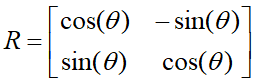t是平移矩阵。在实际问题中，我们知道机器人的里程计Odom (xｏ, yｏ,theta) ! 如果机器人初始位置在地图原点， 那么直接赋值ｔ＝［xｏ, yｏ］
这么赋值的话，就知道了。
ｔ是雷达坐标系原点在地图坐标系中的位置，是雷达坐标系相对于地图坐标系的变换。（即地图坐标系相对于自身怎么平移到与雷达坐标系一致）
那么上面的Ｒ就知道了，R是雷达坐标系在地图坐标系中的姿态，是雷达坐标系相对于地图坐标系的旋转。（即地图坐标系相对于自身怎么旋转与雷达坐标系姿态一致)
＊＊＊右手法则，绕着地图坐标系z轴逆时针旋转为正，那么里程计theta即可直接带入变换矩阵的上式，因为里程计theta就是这么定义的），那么：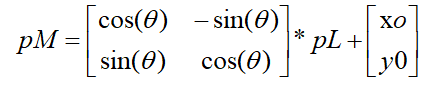2.雷达到地图坐标变换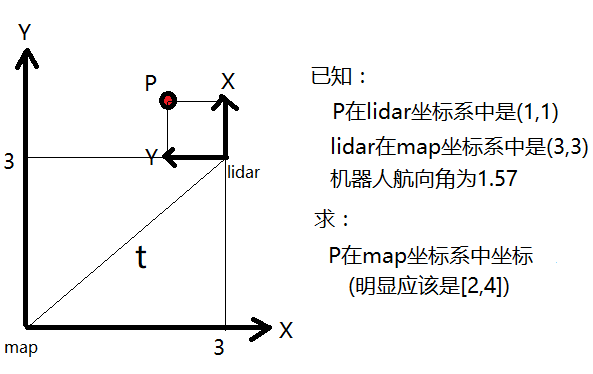已知物体在雷达坐标系坐标(1,1), 机器人里程计数据odom (3, 3, 1,57)，求机器人地图坐标。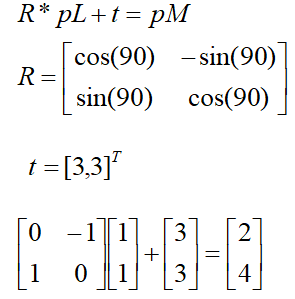这样计算很方便，R使用公式，theta为里程计的角度90，是雷达坐标系相对于map坐标系的旋转变换，是右手定则，map坐标系绕自身z轴逆时针旋转90度。t是雷达坐标系相对于map坐标系的平移变换，即里程计的x和y,是map坐标系相对于自身平移的坐标距离。


展开全文地图坐标系
• 机器人坐标系变换 坐标变换-旋转部分 二维坐标旋转的向量和几何表示 对于以前数学学习不好的人来说，在机器人的坐标变换里，总是各种蒙。 这个图片来给你解答。
• 在SLAM中经常用到空间点的坐标变换。假设已获得相机某一位置的位姿pose，pose包括相机坐标系相对于世界坐标系的旋转R和平移t，此时若已知某点p在相机坐标系下的坐标为Pc，计算点P在世界坐标系下的坐标Pw，可使用 Pw...
在SLAM中经常用到空间点的坐标变换。假设已获得相机某一位置的位姿pose，pose包括相机坐标系相对于世界坐标系的旋转R和平移t，此时若已知某点p在相机坐标系下的坐标为Pc，计算点P在世界坐标系下的坐标Pw，可使用

Pw=R*Pc+t＝T*Pc

开始的时候我有这样的疑问：T表示世界坐标到相机坐标系的变换，而计算的是点坐标从相机坐标系到世界坐标系的变换结果，为什么可以直接乘呢？而不是反向的旋转的平移？其实是因为点的运动和坐标系的运动是正好相反的，所以点坐标乘上反向的变换矩阵是正好可以计算的。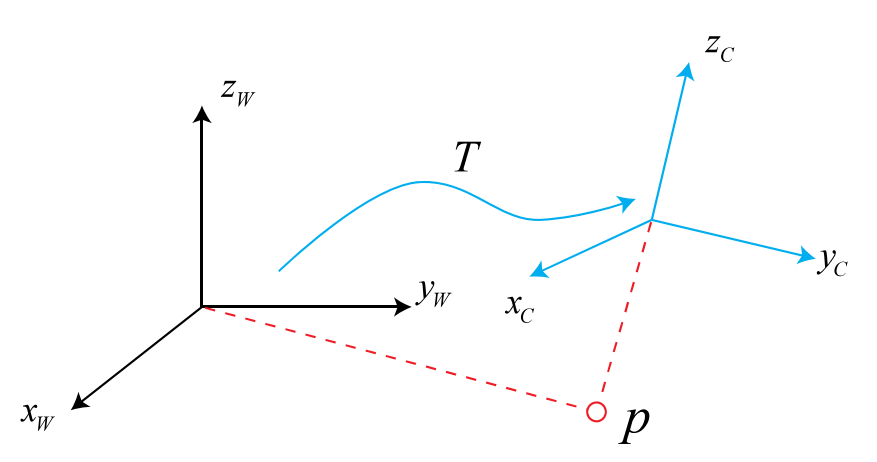展开全文• TF坐标变换 坐标变换是机器人学中一个非常基础，同时也是非常重要的概念。机器人本体和机器人的工作环境中往往存在大量的组件元素，在机器人设计和机器人应用中都会涉及不同组件的位置和姿态，这就需要引入坐标系和...
文章目录TF坐标变换TF功能包TF工具乌龟例程中的TF创建TF广播器创建TF监听器实现乌龟跟随运动存在的问题总结参考
TF坐标变换
坐标变换是机器人学中一个非常基础，同时也是非常重要的概念。机器人本体和机器人的工作环境中往往存在大量的组件元素，在机器人设计和机器人应用中都会涉及不同组件的位置和姿态，这就需要引入坐标系和坐标变换的概念。
对于A，B两个坐标系，A坐标系下的位姿可以通过平移和旋转变换成B坐标系下的位姿，这里的平移和旋转可以通过4×4的变换矩阵来描述。
坐标变换是机器人系统中常用的基础功能，ROS中的坐标变换系统由TF功能包维护。
TF功能包
TF是一个让用户随时间跟踪多个坐标系的功能包，它用树形数据结构，根据时间缓冲并维护多个坐标系之间的坐标变换关系，可以帮助开发者在任意时间，在坐标系间完成点，向量等坐标的变换。

5s之前，机器人头部坐标系相对于全局坐标系的关系是怎样的？
机器人夹取的物体相对于机器人中心坐标系的位置在哪里？
机器人中心坐标系相对于全局坐标系的位置在哪里？

TF可以在分布式系统中进行操作，也就是说，一个机器人系统中的所有坐标变换关系，对于所有的节点组件都是可用的，所有订阅TF消息的节点都会缓冲一份所有坐标系的变换关系数据，这种数据结构不需要中心服务器来存储任何数据。
想要使用TF功能包，总体来说需要以下两个步骤。
（1）监听TF变换
接收并缓存系统中发布的所有坐标变换数据，并从中查询所需要的坐标变化关系。
（2）广播TF变换
向系统中广播坐标系之间的坐标变换关系。系统中可能会存在多个不同部分的TF变换广播，每个广播都可以直接将坐标变换关系插入到TF树中，不需要再进行同步。
TF工具
坐标系统虽然是一个基础理论，但是由于涉及多个空间之间的变换，不容易进行想象，所以TF提供了丰富的终端工具来帮助开发者调试和创建TF变换。
1.tf_monitor
tf_monitor工具的功能是打印TF树中所有坐标系的发布状态，也可以通过输入参数来查看指定坐标系之间的发布状态。
tf_monitor

tf_monitor <source_frame> <target_frame>

2.tf_echo
tf_echo工具的功能是查看指定坐标系之间的变换关系，命令的格式如下：
tf_echo <source_frame> <target_frame>

3.tf_transform_publisher
tf_transform_publisher工具的功能是发布两个坐标系之间的静态坐标变换，这两个坐标系不发生相对位置变化。命令的合适如下：
tf_transform_publisher x y z yaw pitch roll frame_id child_frame_id period_in_ms
tf_transform_publisher x y z qx qy qz qw frame_id child_frame_id period_in_ms

以上两种命令格式，需要设置坐标的偏移参数和旋转参数：偏移参数使用相对于x，y，z三轴的坐标位移；而旋转参数的第一种命令格式使用以弧度为单位的yaw/pitch/roll角度（yaw是围绕z轴旋转的偏航角，pitch是围绕y轴旋转的俯仰角，roll是围绕x轴旋转的翻滚角），第二种命令格式使用四元数表达旋转角度。发布频率以ms为单位。
该命令不仅可以在终端中使用，还可以在launch文件中使用，方法如下：
<launch>
</launch>

4.view_frames
view_frames是可视化的调试工具，可以生成pdf文件，显示整棵TF树的信息。执行方式为：
rosrun tf view_frames

然后使用以下命令，或者pdf阅读器查看生成的pdf文件。
evince frames.pdf

此外，rviz中也提供了TF可视化的插件。
乌龟例程中的TF
接下来我们在乌龟仿真器中通过一个例程（turtle_tf)来理解TF的作用，并且熟悉以上学到的TF工具。该例程的功能包turtle_tf可以使用如下命令进行安装：
sudo apt-get install ros-kinetic-turtle-tf

安装完成后使用如下命令运行例程：
roslaunch turtle_tf turtle_tf_demo.launch

乌龟仿真器打开后会出现两只小乌龟，并且下方的小乌龟会自动向中心位置的小乌龟移动。
打开键盘控制节点，控制中心位置的小乌龟运行：
rosrun turtlesim turtle_teleop_key

另外一只乌龟总会跟随我们控制的那只乌龟运行。在这个例程中，TF是如何运用的呢？首先，使用TF工具来查看以下这个例程中的TF树是什么样的：
rosrun tf view_frames

在当前系统中存在3个坐标系：word，turtle1，tutle2。word是世界坐标系，作为系统的基础坐标系，其他坐标系都相对该坐标系建立，所以word是TF树的根节点。相对于word坐标系，有分别针对两只乌龟创建了两个乌龟坐标系，这两个坐标系的原点就是乌龟在世界坐标系下的坐标位置。
现在要让turtle2跟随turtle1运动，等价于turtle2坐标系要向turtle1坐标系移动，这就需要知道turtle2与turtle1之间的坐标变换。三个坐标系之间的变换关系为：
$T_{turtle1\_turtle2}=T_{turtle1\_word}×T_{word\_turtle2}$
使用tf_echo工具在TF树中查找乌龟坐标系之间的变换关系：
rosrun tf tf_echo turtle1 turtle2

也可以通过rviz的图形界面更加形象地看到这三者之间的坐标关系：
rosrun rviz rviz -d 'rospack find turtle_tf'/rviz/turtle_rviz.rviz

得到turtle2与turtle1之间的坐标变换后，就可以通过计算两只乌龟间的距离和角度，即可控制turtle2向turtle1运动了。
接下来我i们以这个例程为目标，学习如何实现TF的广播和监听功能。
创建TF广播器
首选，我们需要创建一个发布乌龟坐标系与世界坐标系之间TF变换的节点，源码如下：
#include <ros/ros.h>
#include <turtlesim/Pose.h>

std::string turtle_name;

void poseCallback(const turtlesim::PoseConstPtr& msg)
{
// tf广播器

// 根据乌龟当前的位姿，设置相对于世界坐标系的坐标变换
tf::Transform transform;
transform.setOrigin( tf::Vector3(msg->x, msg->y, 0.0) );
tf::Quaternion q;
q.setRPY(0, 0, msg->theta);
transform.setRotation(q);

// 发布坐标变换
br.sendTransform(tf::StampedTransform(transform, ros::Time::now(), "world", turtle_name));
}

int main(int argc, char** argv)
{
// 初始化节点
if (argc != 2)
{
ROS_ERROR("need turtle name as argument");
return -1;
};
turtle_name = argv;

// 订阅乌龟的pose信息
ros::NodeHandle node;
ros::Subscriber sub = node.subscribe(turtle_name+"/pose", 10, &poseCallback);

ros::spin();

return 0;
};

然后使用广播器将坐标变换插入TF树并进行发布，这里发布的TF消息类型是tf::StampedTransform，不仅包含tf::Transform类型的坐标变换、时间戳，而且需要指定坐标变换的源坐标系（parent）和目标坐标系（child）。
创建TF监听器
TF消息广播之后，其他节点就可以监听该TF消息，从而获取需要的坐标变换了。目前我们已经将乌龟相对于world坐标系的TF变换进行了广播，接下来需要监听TF消息，并从中获取turtle2相对于turtle1坐标系的变换，从而控制turtle2移动。源码如下：
#include <ros/ros.h>
#include <tf/transform_listener.h>
#include <geometry_msgs/Twist.h>
#include <turtlesim/Spawn.h>

int main(int argc, char** argv)
{
// 初始化节点
ros::init(argc, argv, "my_tf_listener");

ros::NodeHandle node;

// 通过服务调用，产生第二只乌龟turtle2
ros::service::waitForService("spawn");
node.serviceClient<turtlesim::Spawn>("spawn");
turtlesim::Spawn srv;

// 定义turtle2的速度控制发布器
ros::Publisher turtle_vel =

// tf监听器
tf::TransformListener listener;

ros::Rate rate(10.0);
while (node.ok())
{
tf::StampedTransform transform;
try
{
// 查找turtle2与turtle1的坐标变换
listener.waitForTransform("/turtle2", "/turtle1", ros::Time(0), ros::Duration(3.0));
listener.lookupTransform("/turtle2", "/turtle1", ros::Time(0), transform);
}
catch (tf::TransformException &ex)
{
ROS_ERROR("%s",ex.what());
ros::Duration(1.0).sleep();
continue;
}

// 根据turtle1和turtle2之间的坐标变换，计算turtle2需要运动的线速度和角速度
// 并发布速度控制指令，使turtle2向turtle1移动
geometry_msgs::Twist vel_msg;
vel_msg.angular.z = 4.0 * atan2(transform.getOrigin().y(),
transform.getOrigin().x());
vel_msg.linear.x = 0.5 * sqrt(pow(transform.getOrigin().x(), 2) +
pow(transform.getOrigin().y(), 2));
turtle_vel.publish(vel_msg);

rate.sleep();
}
return 0;
};

该节点首先通过服务调用产生乌龟turtle2，然后声明控制turtle2速度的Publisher。在监听TF消息之前，需要创建一个tf::TransformListener类型的监听器，创建成功后监听器会自动接收TF树的消息，并且缓存10s。然后在循环中就可以实时查找TF树中的坐标变换了，这里需要调用的是tf::TransformListener中的两个接口：

waitForTransform(const std::string &target_frame, const std::string &source_frame, const ros::Time &time, const ros::Duration &timeout)：给定源坐标系和目标坐标系，等待两个坐标系指定时间的变换关系，该函数会阻塞程序运行，因此要设置超时时间。
lookupTransform(const std::string &target_frame, const std::string &source_frame, const ros::Time &time, StampedTransform  &transform)：给定源坐标系和目标坐标系，得到两个坐标系指定时间的变换关系，ros::Time(0)表示我们想要得到最新一次的坐标变换。

通过以上两个接口的调用，就可以获取turtle2相对于turtle1的坐标变换了。然后根据坐标系之间的位置关系，计算得到turtle2需要运动的线速度和角速度，并发布速度控制指令使turtle2向turtle1移动。
实现乌龟跟随运动
编写一个launch文件，使所有节点运行起来，源码：
 <launch>
<!-- 海龟仿真器 -->
<node pkg="turtlesim" type="turtlesim_node" name="sim"/>

<!-- 键盘控制 -->
<node pkg="turtlesim" type="turtle_teleop_key" name="teleop" output="screen"/>

<!-- 两只海龟的tf广播 -->

<!-- 监听tf广播，并且控制turtle2移动 -->
<node pkg="learning_tf" type="turtle_tf_listener"
name="listener" />

</launch>

存在的问题
看理论基本都能明白，但是通过实践，我发现程序并不能实现想要的效果。
启动launch文件后，打开了一个乌龟仿真器，但里面只有一个乌龟，在终端按方向键能控制其运动，但是没有另一只乌龟做跟随运动。
而且在上一个乌龟例程中，乌龟2没有一直跟随乌龟1运动。
这里先记录以下，不知道是程序问题，还是我操作的问题。
总结
通过这个例程的实现，我们学习了TF广播与监听的实现方法，在实际使用中会产生更多的坐标系，TF树的结构也会更加复杂，但是基本的使用方法依然相同。
最近遇到TF的问题，发现对其理解不够深入，所以重温了古月居编写的《ROS机器人开发实践》，这部分内容写得通俗易懂，在此向古月居大佬致敬。
参考
《ROS机器人开发实践》胡春旭 编著
 https://github.com/huchunxu/ros_exploring


展开全文• 前面几节分别介绍了模型变换，视变换，本节从全局把握一遍OpenGL坐标转换的过程，从整体上认识坐标变换过程。 坐标变换的全局图 OpenGL中的坐标处理过程包括模型变换、视变换、投影变换、视口变换等过程，如下图所...OpenGL
• 矢量控制的原理是在永磁同步电机上设法模拟直流电动机的转矩控制规律，经过坐标变换，使其电流矢量分解为产生磁通的电流分量和产生转矩的电流分量，两个分量互相垂直，相互独立。这样就可以对它们进行单独调节，与...永磁同步电机 控制原理
• 弯曲圆环做极坐标变换 被人问到弯曲圆环直接进行极坐标变化会出现什么结果，于是做了个简单的demo； 下面是待处理的图像： 处理后图像： 从正中向上为坐标0度转换结果，明显内圆被压扁，数字已经模糊不可见；因为...计算机视觉
• #极坐标变换就是（x,y）转换为（r,theta）,即（半径，角度） import math import cv2 import numpy as np x=[2,4] center=[4,0] r=math.sqrt(math.pow(x-center,2)+math.pow(x-center,2)) theta=math....
• 坐标变换是空间实体的位置描述，是从一种坐标系统变换到另一种坐标系统的过程。通过建立两个坐标系统之间一一对应关系来实现。
• 坐标变换问题 坐标变换问题分为两种， 一种是物体旋转或平移，坐标系不变，坐标系唯一。应对物体变化的情况 另一种是物体不动，坐标系改变。应对相机变化的情况， ...
• iOS 坐标变换 在iOS中，Quartz 2D提供了坐标变换支持。   一、特殊的坐标变换（平移、缩放、旋转）   1. - void CGContextTranslateCTM ( CGContextRef c, CGFloat tx, CGFloat ty )：平移坐标系统。 该方法相当...
• QT 坐标变换 基本变换 &nbsp;&nbsp;默认的,QPainter在相关设备的坐标系统上进行操作,但是完全支持仿射坐标变换.绘图时可以使用QPainter::scale()函数缩放坐标系统,使用QPainter::rotate()函数顺时针旋转...
• 坐标系统与坐标变换 坐标系统与坐标变换 坐标系统与坐标变换 坐标系统与坐标变换
• 机器人坐标变换 假设有一点P\mathrm{P}P ，在机器人坐标系 (B)\mathrm{(B)}(B) 中其坐标为 PB\mathrm{P^B}PB，在世界坐标系 (A)\mathrm{(A)}(A) 中其坐标为 PA\mathrm{P^A}PA，PA\mathrm{P^A}PA 和 PB\mathrm{P^B}PB...线性代数
• ## 基变换和坐标变换

千次阅读 2019-04-09 10:00:51
12 基变换和坐标变换 基变换和坐标变换 同学们好！大家学完基变换和坐标变换一节后，是不是觉得特别难以理解呢？可是这一节是非常重要的内容哦！坐标变换在解析几何中还有非常重要的应用——就是用来化简二次曲线和...线性代数 线性空间
• 坐标变换学习和理解 1、常见的坐标变换 坐标变换顾名思义是对坐标系进行变换，之所以进行坐标变换是为了问题简化。工程中常用的线性变换主要有Clark变换、Park变换和Dq0变换，其中Clark变换是将ABC三相坐标系变换至...dq变换
• 坐标变换 在平面内选择一个定点O作为“极点”，从该点引出一条射线OX，叫做“极轴”，再选定一个长度单位和角度的正方向(通常取逆时针方向)。对于平面内任何一点M，可以用r来表示OM的长度，用表示OM到OX所转过的...
• ## OpenGL坐标变换

千次阅读 2018-08-16 19:03:52
基础概述 众所周知，OpenGL是一个3D图形库，在终端设备上广泛使用。但是我们的显示设备都是2D平面，那么OpenGL怎么把3D...通过OpenGL坐标变换，我们可以在一个给定的观察视角下，把3D物体投影到2D屏幕上，再经过后...
• 清华大学出版，比较详细的一款三维坐标变换讲解。三维平移变换、比例变换可看成是二维情况的直接推广。但旋转变换则不然，因为我们可选取空间任意方向作旋转轴，因此三维变换处理起来更为复杂。与二维变换相似，我们...
• 坐标系统与坐标变换 第五章 坐标系统与坐标变换 by iwaswzq 2005/1/22 经常有朋友提问关于编程过程中遇到的坐标变换问题。我抽了点时间从msdn摘译了一些东西，并加了一些自己的理解，希望能有助于对程序中...
• 坐标变换或空间变换，本质是相对坐标的变化，绝对坐标没变。 世界空间有两个物体A,B。将A变换到B的坐标空间意思是：将A从世界空间变换到B的局部坐标空间，也就是在B的局部坐标系中重新表示A的坐标（也就是求出A在B...
• ## 过渡矩阵与坐标变换

万次阅读 多人点赞 2016-10-15 08:39:52线性代数...